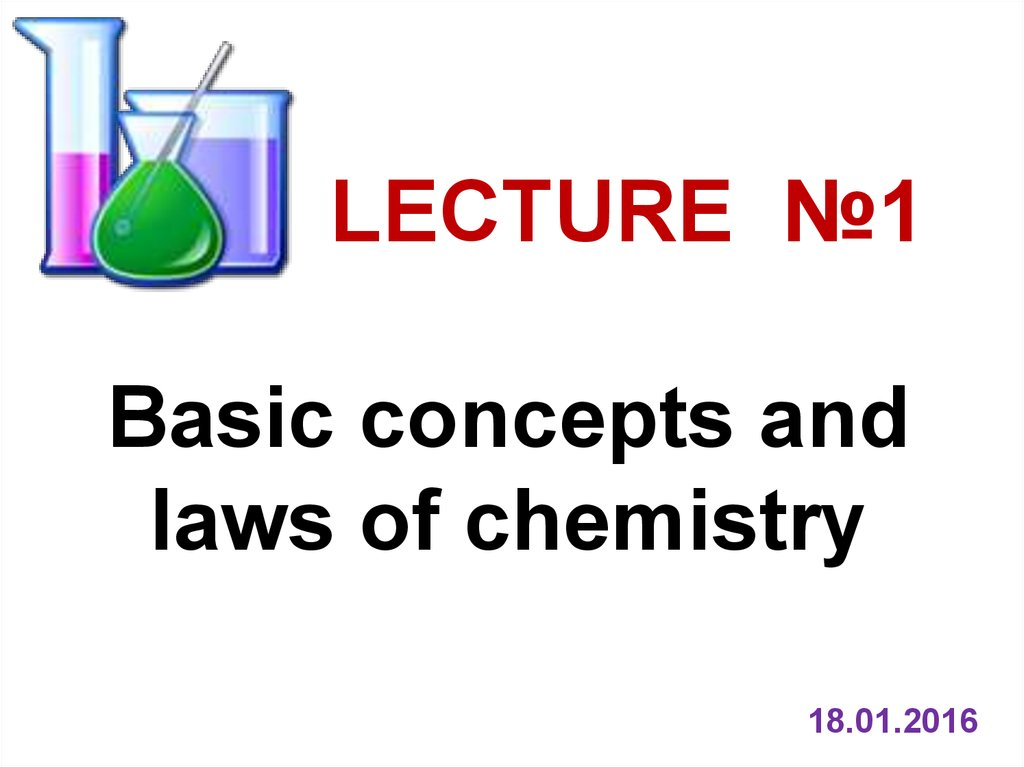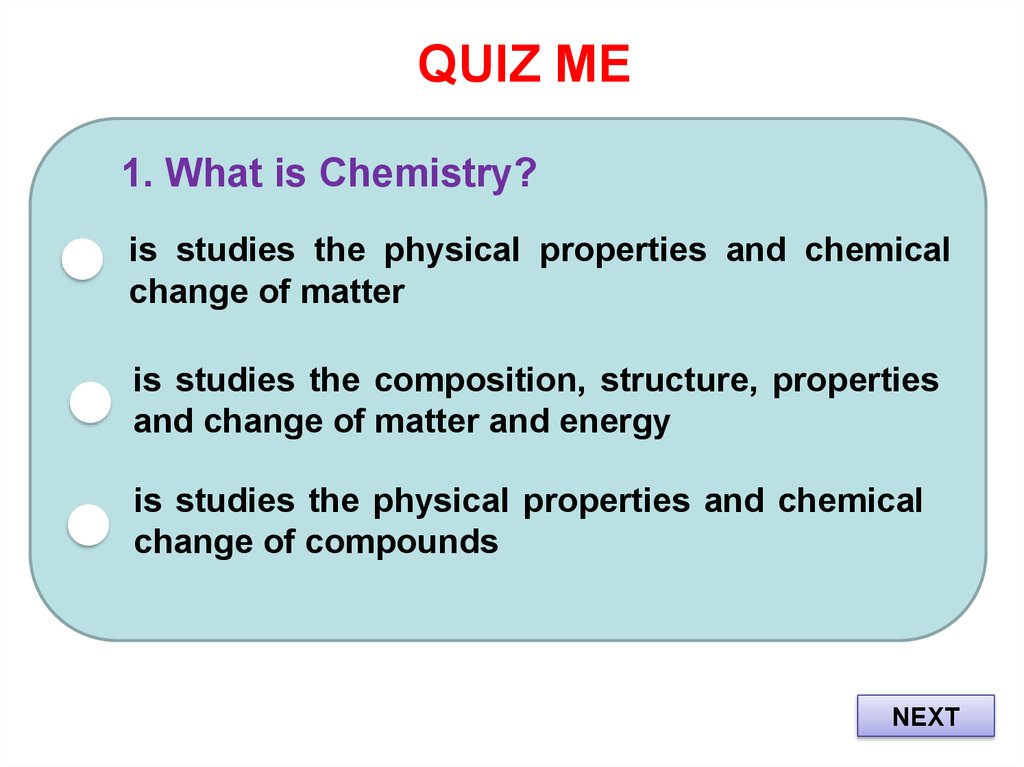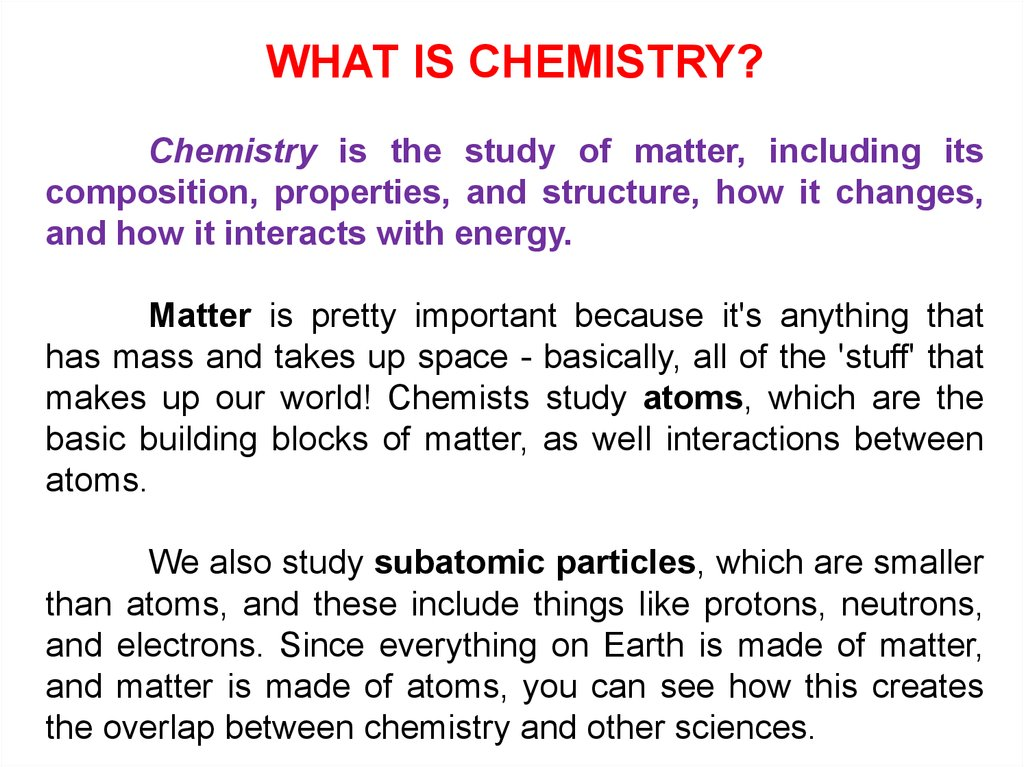# Basic concepts and laws of chemistry

## 1.

LECTURE №1
Basic concepts and
laws of chemistry
18.01.2016

## 2. QUIZ ME

1. What is Chemistry?
is studies the physical properties and chemical
change of matter
is studies the composition, structure, properties
and change of matter and energy
is studies the physical properties and chemical
change of compounds
NEXT

## 3.

WHAT IS CHEMISTRY?
Chemistry is the study of matter, including its
composition, properties, and structure, how it changes,
and how it interacts with energy.
Matter is pretty important because it's anything that
has mass and takes up space - basically, all of the 'stuff' that
makes up our world! Chemists study atoms, which are the
basic building blocks of matter, as well interactions between
atoms.
We also study subatomic particles, which are smaller
than atoms, and these include things like protons, neutrons,
and electrons. Since everything on Earth is made of matter,
and matter is made of atoms, you can see how this creates
the overlap between chemistry and other sciences.

## 4.

The universe is composed of matter and energy.
Matter is anything that occupies space and has rest
mass and volume – rocks, oceans, the air that we breather
and we, ourselves, are all composed of matter.
Energy has no shape or form – it is defined as ability
to do work.
Matter is generally observed in three physical states:
Matter
gas, liquid, solid
Pure substances
separated
is a single pure form of matter
Elements
metals and non-metals
Mixtures
contain more than one substance
reactions
Compounds

He
O2
H2 O
NaCl

## 6.

QUIZ ME
2. A pure substance can only be:
a heterogeneous mixture
an element or a compound
an element
compound

## 7.

A MIXTURE
is any physical material that is made up of various
constituent substances, which haven't chemically
interacted with each other.
A HETEROGENEOUS
MIXTURE
A HOMOGENEOUS
MIXTURE
is a mixture that composes of
components that aren’t uniform
or they have localized regions
that all have different properties.
• Emulsion
• Suspension
• Aerosol
• Smoke
is simply any mixture that is
uniform
in
composition
throughout.
• Precious stones
• Alloys
• Air
• Solution

## 8.

QUIZ ME
3.
Which one of the following mixture is
homogeneous?
starch and sugar
graphite and charcoal
calcium carbonate and calcium bicarbonate
ethanol and water

## 9.

INORGANIC COMPOUNDS
Mineral
acids
Oxides
Bases
And
Alkalis
Salts

## 10.

PHYSICAL STATE OF MATTER
Solid the form of matter characterized by
rigidity;
a
solid
is
relatively
incompressible and has fixed shape and
volume.
Liquid the form of matter that is a
relatively incompressible fluid; a liquid
has a fixed volume but no fixed shape.
Gas the form of matter that is an easily
compressible fluid; a given quantity of
gas will fit into a container of almost any size
and shape.

## 13.

An atom is the basic unit of a chemical element.
Everything in the world is made out of atoms.
An element is a pure substance that cannot be broken
down by chemical methods into simpler components.
Combinations of atoms are called molecules. A
molecule is a part of matter that is made up of more than one
atom. Molecules may contain one kind of atom or more than
one kind of atom.
A compound is a pure substance that consists of two or
more elements chemically combined in a fixed proportion. All
compounds are molecules, but not all molecules are
compounds.
An ion is an atom or group of atoms in which the
number of electron s is different from the number of protons.
Atoms may lose electrons to form cations, or gain electrons to
form anions.

## 14.

A chemical reaction is the change that occurs when
atoms rearrange themselves and form new compounds.
• Combination reaction is a reaction in which two or more
reactant combine to form a single product is known as
combination reaction.
• Decomposition reaction is a reaction in which a single
compound breaks into two or more simpler compounds is
known as decomposition reaction.
• Displacement reaction is a chemical reaction in which a
more reactive element displaces a less reactive element from
its aqueous salt solution.
• Double Displacement reaction is a chemical reaction in
which ions gets exchanged between two reactants which
forms a new compound is called as double displacement
reaction.
• Precipitation Reaction is a chemical reaction that involves
the formation of an insoluble product (precipitate; solid)

## 15.

TYPES OF CHEMICAL REACTIONS

## 17. QUIZ ME

4. The father of modern chemistry is:
Dalton
Lavoisier
Mendeleeff
Proust
NEXT

## 18.

Chemical reactions are governed by certain laws, which
have become fundamental concepts in chemistry. Some of
them are:
• Law of conservation of energy leads to the important concepts
of equilibrium, thermodynamics and kinetics.
• Law of conservation of mass continues to be conserved
in isolated systems, even in modern physics.
• Law of multiple proportions
• Law of definite composition, although in many systems
(notably biomacromolecules and minerals) the ratios tend to require
large numbers, and are frequently represented as a fraction.
• Fick's laws of diffusion
• Le Chatelier's principle
Gas Laws:
• Boyle's law (1662, relating pressure and volume)
• Charles's law (1787, relating volume and temperature)
• Gay-Lussac's law (1809, relating pressure and temperature)
• Henry's law

## 19.

Antoine Lavoisier (1743–1794), a
French chemist, was one of the rst to insist
on the use of the balance in chemical
research. By weighing substances before and
after chemical change, he demonstrated the
law of conservation of mass, which states
that the total mass remains constant
during a chemical change (chemical
reaction):
Matter can be neither created nor
destroyed, though it can be rearranged.
Mass remains constant in an ordinary
chemical change.

2 Hg O2 2 HgO
2*200
+ 32
432
= 2*(200+16)
= 432

## 21.

The Proust's law of definite proportions
states that a chemical compound always contains
exactly the same proportion of elements by mass
and forms the basis of stoichiometry.
An equivalent statement is the law of constant
composition, which states that all samples of a given
chemical compound have the same elemental
composition.
Thus in the formation of compound,
elements are connected with each other in certain
weight ratios:
m(Cu) : m(S) = Ar(Cu) : Ar(S) = 64 : 32 = 2 : 1
In order to obtain copper sulphide (CuS) must
be mixed copper and sulfur powders in a weight ratio
of 2 : 1

## 22.

DALTON'S ATOMIC THEORY
John Dalton (1808) used the Greek concept
of an atom and the laws of definite proportions,
conservation of mass and multiple proportions to give
the atomic theory on scientific basis.
John Dalton (1766 - 1844)
Dalton proposed that the properties of elements differ from
one another because their atoms differ. He also recognized that
even though they may share the same atoms, compounds have
properties that bear no relationship to those elements of which
they are composed. Dalton’s atomic theory stated that:
1) All matter is made of atoms. Atoms are indivisible and
indestructible.
2) All atoms of a given element are identical in mass and
properties
3) Compounds are formed by a combination of two or
more different kinds of atoms.
4) A chemical reaction is a rearrangement of atoms.

## 23.

An atom is the smallest unit of matter that defines the
chemical elements. Every solid, liquid, gas, and plasma is
made up of neutral or ionized atoms. Atoms are very small:
the size of atoms is measured in picometers - trillionths (10−12)
of a meter.
The atomic mass (ma) is the mass of an atomic particle,
sub-atomic particle, or molecule. The protons and neutrons
account for almost all of the mass of an atom. By international
agreement, 1 unified atomic mass unit is defined as 1/12 of
the mass of a single carbon-12 atom (at rest):
1
27
1 a.m.u. mC 1,66057 10 kg
12
The mass number should also not be confused with the relative atomic mass (also
called atomic weight) of an element, which is the ratio of the average atomic mass of the
different isotopes of that element (weighted by abundance) to the unified atomic mass unit. This
weighted average can be quite different from the near-integer values for individual isotopic
masses.

## 24.

RELATIVE ATOMIC MASS OF AN ELEMENT
For example, there are two main isotopes of chlorine:
35
17
Number of electrons (Z)
Number of protons (Z)
Number of neutrons (A – Z)
Cl
37
17
17
17
18
17
17
20
Cl
In any given sample of chlorine that has not been subjected to
mass separation there will be roughly 75% of chlorine atoms
which are chlorine-35 and only 25% of chlorine atoms which
are chlorine-37. This gives chlorine a relative atomic mass
of 35.5 (actually 35.4527 g/mol).

## 26.

STRUCTURE OF CARBON ATOMS
Mass number = 6 protons + 6 neutrons
Ar=12
It is the total number of
protons
and
neutrons
present in the nucleus of an
atom.
Atomic number = 6 protons / 6 electrons
12,011
ATOMIC WEIGHT
Z=6
The number of protons
present in the nucleus of an
atom is called the atomic
number.

## 28.

Hydrogen has three isotopes:
1 proton
1 proton
1 proton
0 neutrons
1 neutron
2 neutrons

## 30.

CHEMICAL BOND
The sharing or transfer of electrons creates some attraction force
between elements that is called as chemical bond.
Types of Chemical Bonding:
As a matter of convenience we usually divide chemical bonds into
different types. There are two major classes of bonding:
Ionic bonding which results from electrostatic interaction among ions;
and can be formed by the transfer of one or more electrons from one atom or
group of atoms to another.
Covalent bonding which results from sharing one or more electron
pairs between two atoms.
These represent two extremes and all bonds have at least some
degree of both ionic and covalent character. Compounds in which the bonding
is predominantly ionic are called ionic compounds, and those in which the
bonding is predominantly covalent are called covalent compounds.
Bonding between metal ions is known as metallic bonding.
A hydrogen bond is an interaction that directs the association of the
covalently bounded hydrogen atom with one or more other atoms, group of
atoms or molecules into an aggregate structure that is sufficiently stable to
make it convenient for the chemist to consider it as an independent chemical
species.

## 31.

The amount of a substance is the mole (symbol:
mol) is defined arbitrarily as the amount of a substance
which has as many atoms or molecules as there are
atoms in 12 grams of the carbon isotope C-12.
The number of atoms in a mole is called
Avogadro's number, the value of which is
approximately 6.022 × 1023.
One mole of a substance always contains
almost exactly the relative atomic mass or molar mass
of that substance.
mA
for atom :
Ar
mM
for compound :
Mr
N
N
N A 6,02 10 23

## 32.

Relative molecular mass (Mr) or molecular weight
refers to the mass of a molecule is calculated as the sum of the
mass of each constituent atom multiplied by the number of
atoms of that element in the molecular formula:
Mr ( Na2 SO4 ) 23 2 32 1 16 4 142 m.m.u
In chemistry, the molar mass M is a physical
property. It is defined as the mass of a given substance
(chemical element or chemical compound) divided by it’s
a amount of substance. The base SI unit for molar mass is
kg/mol. However, molar masses are almost always
expressed in g/mol. As an example, the molar mass of
sodium sulfate is approximately:
M ( Na2 SO4 ) 142 g / mol

## 33.

Even before the creation of the doctrine of atom
and molecula it was found that simple and complex
chemical substances react in the reaction mass in
strictly defined ratios.
Law of equivalents: All substances react and
form in equivalent proportions.
The equivalent ratio is the same number of
moles of equivalents. Thus the law of equivalents
can be formulated differently: the number of mole
equivalents for all substances involved in the
reaction is the same.

## 34.

The equivalent (symbol: Eq), sometimes
termed the molar equivalent, is a unit of electrical
charge used in chemistry.
The equivalent of substance A is the amount
of substance A multiplied by its valence.
EqElement
Ar
Val
Ar ( Al ) 27
for metal Eq( Al )
9 g eq / mole
Val ( Al ) 3
Ar (O ) 16
for non metal Eq(O)
8 g eq / mole
Val (O) 2

## 35.

The equivalent could be also formally defined
through the amount of substance which will either: react
with or supply one mole of hydrogen ions (H+) in an acidbase reaction; or react with or supply one mole of
electrons in a redox reactiom.
The mass of one equivalent of a substance is called
its equivalent weight.
7
1
Eq( H 2 S )
2
1
Eq( NH 3 )
3
1
Eq(CH 4 )
4
5 e 2
K Mn O4 H 2 SO4 Mn SO4 H 2O
EqOX ( KMnO4 )
4
2 e
1
e
1
5e
6
Na2 S O3 Na2 S O4
EqRED ( Na2 SO3 )
1
e
1
2e

## 36.

Eq(acid H X EOY )
M H x EOy
x( H )
M Me( OH ) n
Eq(base Me(OH ) n )
n(OH )
II
M E X OY
Eq(oxide E X OY )
2 Y (O)
M MeX EOY
Eq( salt Me X EOY )
X Me Val Me

## 37.

Law of Equivalents: the mass ratio
of the reacting and produced
substances in the reaction is directly
proportional to the ratio of their
equivalent weights
m A Eq A
m B Eq B
mA
Eq A
or for gas :
V gas EV ( gas)

## 38.

Amedeo
introduced
the
term "molecule" and distinguished it from 'atom'.
According to Avogadro, particles in the gaseous
state do not exist as atoms but as molecules.
In 1811 he proposed his famous hypothesis, now known
as 'Avogadro's Law'. The Law states that "Equal volume of all the
gases at same temperature and pressure, contains equal
number of molecules."
• Standard temperature and pressure is called as STP. For gases,
the term STP is often used. STP means the temperature of the gas is
273K and the pressure of the gas is 1 atm. Avogadro said that 1
mole of any gas at STP occupies 22.4L of volume.
• Avogadro also expressed the number of atoms present in the
mole of a gas. He stated that 6.022 x 1023 particles are present in
the 1 mole of a gas.

## 39.

BOYLE’S LAW
According to Boyle’s Law when
the temperature is constant, the
pressure and volume of a gas are
inversely proportional (PV = constant)
P1V1 = P2V2
Thus increasing the gas pressure, it s
volume is reduced.

## 40.

CHARLES’ LAW
According to Charles’ Law the
volume of a gas is proportional to the
Kelvin temperature as long as the
pressure is constant (V = k*T)
V1 V2
T1 T2
Note: The temperature for gas laws must always be
expressed in Kelvin where Kelvin = oC +273.15

## 41.

GAY-LUSSAC'S LAW
Gay Lussac's Law of pressure and
temperature describes the direct relationship
between pressure and temperature, if mass and
volume of gas are fixed. If the absolute
temperature of a fixed gas volume is
increased, then the pressure will be
increases proportionally (P=k*T):
P1 P2
T1 T2

## 42.

Partial Pressure
Pressure each gas in a mixture would
exert if it were the only gas in the container:
PA V A P Vgases mixture P
'
A
'
A
PA V A
Vgases mixture
Dalton's Law of Partial Pressures (1801)
The total pressure exerted by a gas
mixture is the sum of the partial pressures
(P n) of the gases in that mixture:
PTotal = P 1 +
P 2 + P 3 + .....

## 43.

Dalton’s Law of Partial Pressures
V=const
V=const
P А
P В
V=const
Ptotal = P А + P В

## 44.

THE COMBINED GAS LAW
If the amount of the gas is constant,
then Boyle’s Charles’ and Gay-Lussac’s
Laws can be combined into one
relationship:
P1 V1 P2 V2
T1
T2